There are many ways to compute navigation functions. The cost-to-go function determined by Dijkstra's algorithm working backward from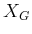yields an optimal navigation function. The third condition of a navigation function under the anisotropic case is exactly the stationary dynamic programming equation, (2.18), if the navigation function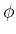is defined as the optimal cost-to-go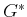. It was mentioned previously that the optimal actions can be recovered using only the cost-to-go. This was actually an example of using a navigation function, and the resulting procedure could have been considered as a feedback plan.
If optimality is not important, then virtually any backward search algorithm from Section 2.2 can be used, provided that it records the distance to the goal from every reached state. The distance does not have to be optimal. It merely corresponds to the cost obtained if the current vertex in the search tree is traced back to the root vertex (or back to any vertex in, if there are multiple goal states).
If the planning problem does not even include a cost functional, as in Formulation 2.1, then a cost functional can be invented for the purposes of constructing a navigation function. At each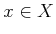from whichis reachable, the number of edges in the search graph that would be traversed fromtocan be stored as the cost. If Dijkstra's algorithm is used to construct the navigation function, then the resulting feedback plan yields executions that are shortest in terms of the number of stages required to reach the goal.
The navigation function itself serves as the representation of the feedback plan, by recovering the actions from the local operator. Thus, a function,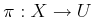, can be recovered from a navigation function,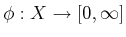. Likewise, a navigation function,, can be constructed from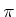. Therefore, theandcan be considered as interchangeable representations of feedback plans.